# Sliding motion along a frictionless inclined plane

(diff) ← Older revision | Latest revision (diff) | Newer revision → (diff)
This article discusses a scenario/arrangement whose statics/dynamics/kinematics can be understood using the ideas of classical mechanics.
View other mechanics scenarios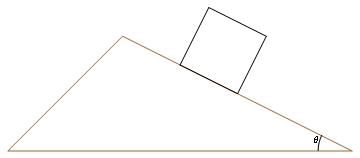The brown triangle is the fixed inclined plane and the black block is placed on it with a dry frictionless surface of contact.

The scenario here is a dry block (with a stable surface of contact) on a dry fixed frictionless inclined plane, with$\theta$ being the angle of inclination with the horizontal axis.

The extremes are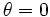$\theta = 0$ (whence, the plane is horizontal) and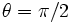$\theta = \pi/2$ (whence, the plane is vertical).

Though the picture depicts a square cross section, this shape assumption is not necessary.

A more general scenario that includes the case of an inclined plane with friction is sliding motion along an inclined plane.

## Basic components of force diagram

A good way of understanding the force diagram is using the coordinate axes as the axis along the inclined plane and normal to the inclined plane. For simplicity, we will assume a two-dimensional situation, with no forces acting along the horizontal axis that is part of the inclined plane (in our pictorial representation, this no action axis is the axis perpendicular to the plane).

### The two candidate forces

Assuming no external forces are applied, there are four candidate forces on the block:

Force (letter) Nature of force Condition for existence Magnitude Direction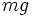$mg$ gravitational force unconditional$mg$ where$m$ is the mass and$g$ is the acceleration due to gravity vertically downward, hence an angle$\theta$ with the normal to the incline and an angle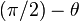$(\pi/2) - \theta$ with the incline$N$ normal force unconditional adjusts so that there is no net acceleration perpendicular to the plane of the incline outward normal to the incline

### Taking components of the gravitational force

The most important thing for the force diagram is understanding how the gravitational force, which acts vertically downward on the block, splits into components along and perpendicular to the incline. The component along the incline is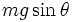$mg\sin \theta$ and the component perpendicular to the incline is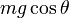$mg\cos \theta$. The process of taking components is illustrated in the adjacent figure

### Component perpendicular to the inclined plane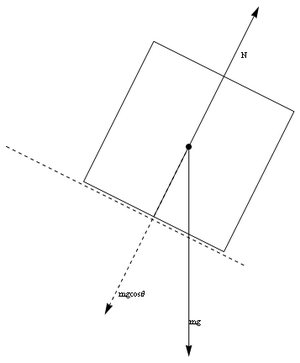Normal component$mg\cos \theta$ of gravitational force$mg$ should cancel out normal force.

In this case, assuming a stable surface of contact, and that the inclined plane does not break under the weight of the block, and no other external forces, we get the following equation from Newton's first law of motion applied to the axis perpendicular to the inclined plane: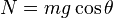$\! N = mg \cos \theta$

where$N$ is the normal force between the block and the inclined plane,$m$ is the mass of the block, and$g$ is the acceleration due to gravity.$N$ acts inward on the inclined plane and outward on the block. Some observations:

Value/change in value of$\theta$ Value of$N$ Comments$\theta = 0$ (horizontal plane)$N = mg$ The normal force exerted on a horizontal surface equals the mass times the acceleration due to gravity, which we customarily call the weight.$\theta = \pi/2$ (vertical plane)$N = 0$ The block and the inclined plane are barely in contact and hardly pressed together.$\theta$ increases from$0$ to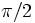$\pi/2$$N$ reduces from$mg$ to$0$. The derivative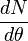$\frac{dN}{d\theta}$ is$-mg\sin \theta$ The force pressing the block and the inclined plane reduces as the slope of the incline increases.

For simplicity, we ignore the cases$\theta = 0$ and$\theta = \pi/2$ unless specifically dealing with them.

### Component along (down) the inclined plane

For the axis down the inclined plane, the gravitational force component is$mg\sin \theta$. There are no other forces in this direction, so, if$a$ denotes the acceleration measured positive in the downward direction, we get: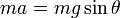$ma = mg \sin \theta$

After cancellation of$m$, we get:$a = g \sin \theta$

Note that if the block is sliding upward (for instance, if given an initial upward velocity) this acceleration functions as retardation, whereas if the block is sliding downward (which may happen if the block is placed at rest, or given an initial downward velocity, or of it turns back after sliding upward) then the acceleration increases the speed.

## Behavior for a block initially placed at rest

### Kinematics

The kinematic evolution in the second case is given as follows, if we set$t = 0$ as the time when the block is placed, we have the following (here, the row variable is written in terms of the column variable):$\! v$$\! t$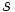$\! s$ vertical displacement (call$\!h$) horizontal displacement (call$\! x$)$\! v$$\! v$$\! gt\sin \theta$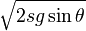$\! \sqrt{2sg\sin \theta}$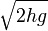$\! \sqrt{2hg}$$\! \sqrt{2xg\tan \theta}$$\! s$$\! v^2/(2g\sin \theta)$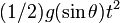$\! (1/2)g (\sin \theta) t^2$$\! s$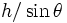$\! h/\sin \theta$$\! x/\cos \theta$$\! h$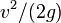$\! v^2/(2g)$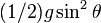$\! (1/2) g \sin^2\theta$$\! s \sin \theta$$\! h$$\! x \tan \theta$$\! x$$\! v^2/(2g\tan \theta)$$\! (1/2)g \cos \theta \sin \theta$$\! s \cos \theta$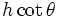$\! h \cot \theta$$\! x$